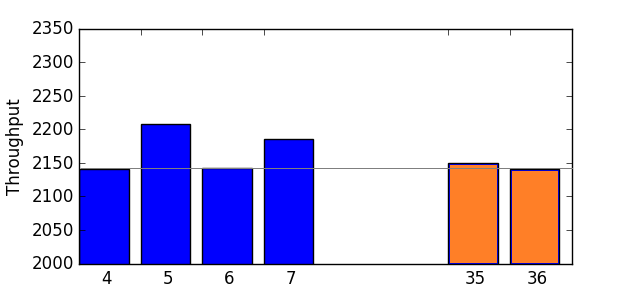# Paxos Performance Modeling – Part 1.5

This post is a quick update/conclusion to the part 1. So, does the network variations make any impact at all? In the earlier simulation I showed some small performance degradation going from 3 to 5 nodes.

The reality is that for paxos, network behavior makes very little difference on scalability, and in some cases no difference at all. To see what I mean, look at the figure below:See how 4 and 6 and 36 node perform the same in my simulation? And how 5, 7 and even 35 nodes clusters slightly outperforms 4 nodes?

The intuitive high level explanation for even-numbered simulation results is quite simple. For even numbered cluster sizes, a round leader receives an odd number of replies, assuming a self-voting leader. The leader also decides the round after reaching a majority  quorum $$q=\left \lfloor{\frac{N}{2}}\right \rfloor +1$$, meaning that it needs to receive $$q-1$$  or $$\left \lfloor{\frac{N}{2}}\right \rfloor$$messages (with self-voting). As it happens, for even clusters, this message is exactly the median fastest message of the round. For instance, a 6 node cluster leader will receives 5 replies, but the round reaches the majority at the 3rd (or median) reply.

Since the simulation draws message RTTs from a normal distribution, the median (50th  percentile) RTT is also the mean. After repeating it for sufficient number of rounds, any fluctuations are averaged out, resulting in an average round decided by a message with an average RTT for the network.

The cluster with odd number of servers, however, decides on the round at a message with RTT slightly less then the median RTT. This is because we have an even number of replies, and median is computed be averaging two middle RTTs. The smaller of the two values used for computing the median is actually the quorum message for the round.  For instance, in a 7-node deployment, the leader reaches quorum after receiving 3rd message $$l_3$$, with median being $$\frac{l_3+l_4}{2}$$

As a result, after many round repeats (I do ~125000 rounds) the simulation still ends up with an average RTT of a quorum message to be a tiny bit less than the median/mean RTT, and the more nodes I add, the closer it becomes to the actual 50th percentile and the mean.

So, what do we have after all of this? I think it is safe to assume the effects of network variance on paxos performance are very small and sometimes non-existent. We should not worry about the network as much, as long as it is stable and delivers predictable performance.  However, if you have a system with non-majority quorums, you may get slight benefit from quicker replies.

Update (3/10/2018):

• Part 2 – Queuing/Processing overheads